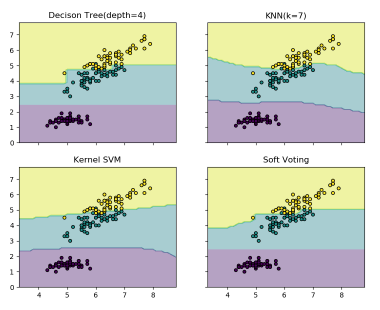# 【深度学习】解析深度学习的集成方法1人已赏
Fizz 发布于 2021-06-15 17:25:42 浏览 3053 点赞 93 收藏 7

## 【深度学习】解析深度学习的集成方法``````文章目录
1 前言
2 使用集成模型降低方差
3 如何集成神经网络模型
3.1 Varying Training Data
3.2 Varying Combinations
3.3 总结
4 深入了解模型融合Ensemble(深度+代码)
4.1 Voting ensembles（投票）
4.2 averaging（平均）
5 集成学习的投票机制（Voting mechanism about ensemble learning）``````

# 3 如何集成神经网络模型

The oldest and still most commonly used ensembling approach for neural networks is called a “committee of networks.” 通常选择多模型的方式：多个相同配置的神经网络 + 相同的训练数据集 + 不同的参数随机初始化 集成的模型数量通常比较小，原因如下： 考虑计算复杂度。 当模型数目达到一定程度，随着数目的增加，集成模型得到的性能回报变小。 集成模型一般考虑如下三种构造方式： Trainning Data: 不同单模型使用不同的训练数据 Ensemble Models: 选择不同的单模型 Combinations: 选择不同的组合方式

## 3.3 总结

``````Varying Training Data
k-fold Cross-Validation Ensemble
Bootstrap Aggregation (bagging) Ensemble
Random Training Subset Ensemble
Varying Models
Multiple Training Run Ensemble
Hyperparameter Tuning Ensemble
Snapshot Ensemble
Horizontal Epochs Ensemble
Vertical Representational Ensemble
Varying Combinations
Model Averaging Ensemble
Weighted Average Ensemble
Stacked Generalization (stacking) Ensemble
Boosting Ensemble
Model Weight Averaging Ensemble``````

# 4 深入了解模型融合Ensemble(深度+代码)

## 4.1 Voting ensembles（投票）

``样本：1111111111``

``````A: 1111111100 = 80% accuracy
B: 0111011101 = 70% accuracy
C: 1000101111 = 60% accuracy``````

``Z: 1111111101 = 90% accuracy``

2.选择弱相关性的融合 同上，10个样本，真实数据都是1。通过模型A，B，C得到准确度分别为80%，80%，70%的结果（单模型效果明显比之前的模型们要好），但是三个模型高度相关。通过majority vote得到结果Z：

``````样本：1111111111
A: 1111111100 = 80% accuracy
B: 1111111100 = 80% accuracy
C: 1011111100 = 70% accuracy
Z: 1111111100 = 80% accuracy``````

## 4.2 averaging（平均）

Id,Prediction 1,0.35000056 2,0.35000002 3,0.35000098 4,0.35000111 模型2：

Id,Prediction 1,0.57 2,0.04 3,0.99 4,0.96 模型1和模型2直接averaging融合的话，模型1几乎对模型2没有影响，就是我们刚才说的波动较小的模型在融合过程中起的作用较小的问题。 我们采用Rank Averaging这种方式，则有：

Id,Prediction 1,0.33 2,0.0 3,0.83 4,0.83

# 5 集成学习的投票机制（Voting mechanism about ensemble learning）

``````#训练多数规则分类器：
from sklearn import datasets
from sklearn.model_selection import cross_val_score
from sklearn.linear_model import LogisticRegression
from sklearn.naive_bayes import GaussianNB
from sklearn.ensemble import RandomForestClassifier
from sklearn.ensemble import VotingClassifier

X,y = iris.data[:,1:3],iris.target
clf1 = LogisticRegression(solver='lbfgs',multi_class='multinomial',random_state=1)
# solver:逻辑回归损失函数的优化方法,拟牛顿法的一种。利用损失函数二阶导数矩阵即海森矩阵来迭代优化损失函数。
clf2 = RandomForestClassifier(n_estimators=50,random_state=1)
clf3 = GaussianNB()
eclf = VotingClassifier(estimators=[('lr',clf1),('rf',clf2),('gnb',clf3)],voting='hard')
for clf,label in zip([clf1,clf2,clf3,eclf],['Logistic Regression','Random Forest','Naive Bayes','Ensemble']):
scores = cross_val_score(clf,X,y,cv=5,scoring='accuracy')
print("Accuracy:均值：%0.2f，标准差:%0.2f [%s]" %(scores.mean(),scores.std(),label))``````

``````from sklearn import datasets
import numpy as np
from sklearn.tree import DecisionTreeClassifier
from sklearn.neighbors import KNeighborsClassifier
from sklearn.svm import SVC
from itertools import product
from sklearn.ensemble import VotingClassifier
import matplotlib.pyplot as plt

X,y = iris.data[:,[0,2]],iris.target
#???data[:,[0,2]]
#Training
clf1 = DecisionTreeClassifier(max_depth=4)
clf2 = KNeighborsClassifier(n_neighbors=7)
clf3 = SVC(gamma='scale',kernel='rbf',probability=True)
#gamma:核函数系数
# kernel:算法中采用的核函数类型,‘rbf’：径像核函数/高斯核
# probability:是否启用概率估计
eclf = VotingClassifier(estimators=[('dt',clf1),('knn',clf2),('svc',clf3)],
voting='soft',weights=[2,1,2])
clf1 = clf1.fit(X,y)
clf2 = clf2.fit(X,y)
clf3 = clf3.fit(X,y)
eclf = eclf.fit(X,y)

# Plotting decision regions
x_min,x_max = X[:,0].min() - 1,X[:,0].max() + 1
y_min,y_max = X[:,1].min() - 1,X[:,1].max() + 1
xx,yy = np.meshgrid(np.arange(x_min, x_max, 0.1),np.arange(y_min, y_max, 0.1))
# 生成网格点坐标矩阵:
# 坐标矩阵——横坐标矩阵XX中的每个元素，与纵坐标矩阵YY中对应位置元素，共同构成一个点的完整坐标。
# 如B点坐标(X12,Y12)=(1,1)(X12​,Y12​)=(1,1)
f, axarr = plt.subplots(2, 2, sharex='col', sharey='row', figsize=(10, 8))
# sharey‘row’ 时，每一行的子图会共享 x 或者 y 轴
for idx,clf,tt in zip(product([0,1],[0,1]),
[clf1,clf2,clf3,eclf],
['Decison Tree(depth=4)','KNN(k=7)','Kernel SVM','Soft Voting']):
Z = clf.predict(np.c_[xx.ravel(),yy.ravel()])
'''
numpy中的ravel()、flatten()、squeeze()都有将多维数组转换为一维数组的功能，区别：
ravel()：如果没有必要，不会产生源数据的副本
flatten()：返回源数据的副本
squeeze()：只能对维数为1的维度降维
另外，reshape(-1)也可以“拉平”多维数组
'''
'''
np.r_ 是按列连接两个矩阵，就是把两矩阵上下相加，要求列数相等，类似于 pandas 中的 concat()。
np.c_ 是按行连接两个矩阵，就是把两矩阵左右相加，要求行数相等，类似于pandas中的merge()。
'''
Z = Z.reshape(xx.shape)
axarr[idx, idx].contourf(xx, yy, Z, alpha=0.4)
# contourf绘制等高线的，contour和contourf都是画三维等高线图的，不同点在于contour() 是绘制轮廓线，contourf()会填充轮廓
axarr[idx, idx].scatter(X[:, 0], X[:, 1], c=y,
s=20, edgecolor='k')
axarr[idx, idx].set_title(tt)
plt.show()``````*本文仅代表作者观点，不代表易百纳技术社区立场。系作者授权易百纳技术社区发表，未经许可不得转载。

### 相关专栏海思讨论群君正智能视频群客服微信： x460910098客服手机： 17714209983